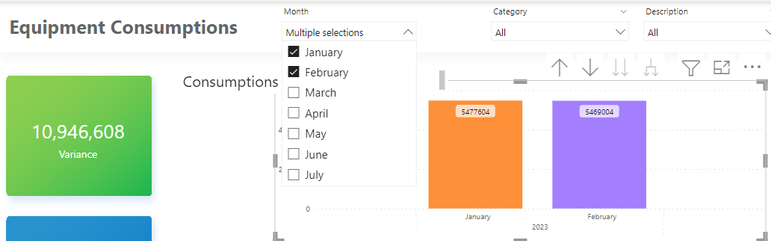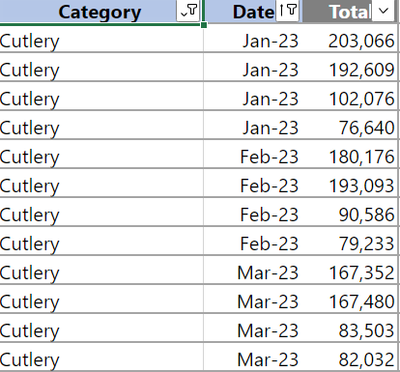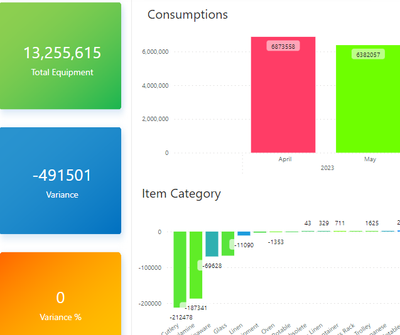cancel
Showing results for
Did you mean:Frequent Visitor

## Variance between filtered date

Hi, How to get the variance for filtered dates.

example :

 Date Total Jan 5477604 Feb 5469004 Variance -8,6001 ACCEPTED SOLUTIONSuper User

Variance =
VAR MaxMonth =
MAX ( MonthsF[YearMonth] )
VAR MinMonth =
MIN ( MonthsF[YearMonth] )
VAR MaxMonthValue =
CALCULATE ( SUM ( Consumptions[Total] ), MonthsF[YearMonth] = MaxMonth )
VAR MinMonthValue =
CALCULATE ( SUM ( Consumptions[Total] ), MonthsF[YearMonth] = MinMonth )
RETURN
MaxMonthValue - MinMonthValue

9 REPLIES 9Frequent Visitor

Hi, I have a Date table shown below.

however I don't know how to get the variance. I'm using only one column for date and I want to select between months. please helpSuper User

Variance =
VAR MaxMonth =
MAX ( MonthsF[YearMonth] )
VAR MinMonth =
MIN ( MonthsF[YearMonth] )
VAR MaxMonthValue =
CALCULATE ( SUM ( Consumptions[Total] ), MonthsF[YearMonth] = MaxMonth )
VAR MinMonthValue =
CALCULATE ( SUM ( Consumptions[Total] ), MonthsF[YearMonth] = MinMonth )
RETURN
MaxMonthValue - MinMonthValueFrequent VisitorSuper User

@jayceeb
Do yu mean something like this?

``````Variance % =
VAR MaxMonth =
MAX ( MonthsF[YearMonth] )
VAR MinMonth =
MIN ( MonthsF[YearMonth] )
VAR MaxMonthValue =
CALCULATE ( SUM ( Consumptions[Total] ), MonthsF[YearMonth] = MaxMonth )
VAR MinMonthValue =
CALCULATE ( SUM ( Consumptions[Total] ), MonthsF[YearMonth] = MinMonth )
RETURN
DIVIDE ( MaxMonthValue - MinMonthValue, MaxMonthValue )``````Frequent Visitor

Hi. I tried but all shows zero %.Super User

@jayceeb
Change the data type to %.
However, this gives you the percentage difference between the two selected months if this is what you want.Frequent Visitor

OMG. Thanks master. 😅🙏Frequent Visitor

It works. Thank you very much 😭Super User

Hi @jayceeb
I suppose you don't have a date table and the month shown in the visual and the slicer is coming the automatic time intelligence hierarchy?